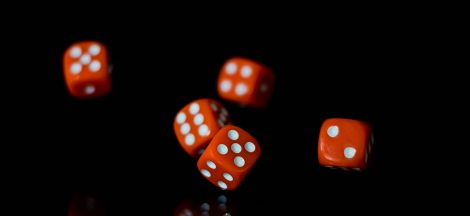As we draw closer to the end of our first semester, I decided to begin my revision of the courses. This is necessary because we are going to end the semester with examinations. My revision of the topics we have done so far in the Data analytics course has helped me to understand the course better.

One of the concepts I revised is the Relative frequency method. This is one of the three methods we can use to assign a probability. Relative frequency is a method of assigning probability based on experimentation or historical data. It is based on the frequency of realization. The division method is used to apply relative frequency. That is, we divide the total points by the frequency of each data point.

In the world of business, we assign a probability to possible outcomes with the use of the relative frequency method. The other method, the classical method, works when we have equally likely outcomes. As this rarely happens in business, managers use the relative frequency method to assign a probability. We can expect this, given that managers deal with a lot of uncertainty.

I have noticed that in the Data Analytics course, one concept leads to the other. And I realized when if I do not understand the preceding topics or concepts, I may struggle with newer ones. Hence, before I got into the relative frequency method, I went over some concepts. These are Experiment, Sample space, Sample point. An experiment is any process that generates well defined outcomes. A sample space is a part of an experiment; it is a set of the experimental outcomes. A sample point is an outcome. Let me use an example to further explain this.

An example of an experiment is to toss a coin. The possible outcomes of this experiment are a head and a tail. These outcomes make up the sample space for this experiment. There are two sample points in the sample space – head, tail.

It is amazing what a revision can do. Going over the concepts have helped me.

I also looked at a concept called Events.

Have you heard of events? Well this is not the regular events we organize; this is event in data analytics (chuckle). So what is an event?

Well, I am happy to tell you that an event in Data analytics is a collection of sample points. For example, a sample space for rolling a die has 6 sample points 1, 2, 3, 4, 5, and 6. We can partition the sample space, that is, we can create a section of the sample space for even numbers and another section for odd numbers. We call each part a subset of the sample space. Each sub-set is called an Event.

We are sure to come across events when we want to assign probability. If we have an experiment, example the rolling of a die, we identify the sample points which make up the sample space – 1, 2, 3, 4, 5, and 6. We can partition the sample space into two (2) subsets – even numbers and odd numbers. Remember we call each subset an event.

In light of the above, the probability of an event (a subset) is equal to the sum of the sum of the individual probabilities of the sample points in the event.

Let me use numbers to illustrate this:

Event (even numbers) – 2, 4, 6

The probability of each sample points

2 = 1/6

4 = 1/6

6 = 1/6

Total = 3/6 break down to 1/2

So what do think? The probability of the event is 1/2.

Now that I can do this on my own, I will continue my revision of the rest of the topics.## My BLOGGING EXPERIENCE AS AN AID TO BETTER MY WRITING SKILL

in## EXAMS

in